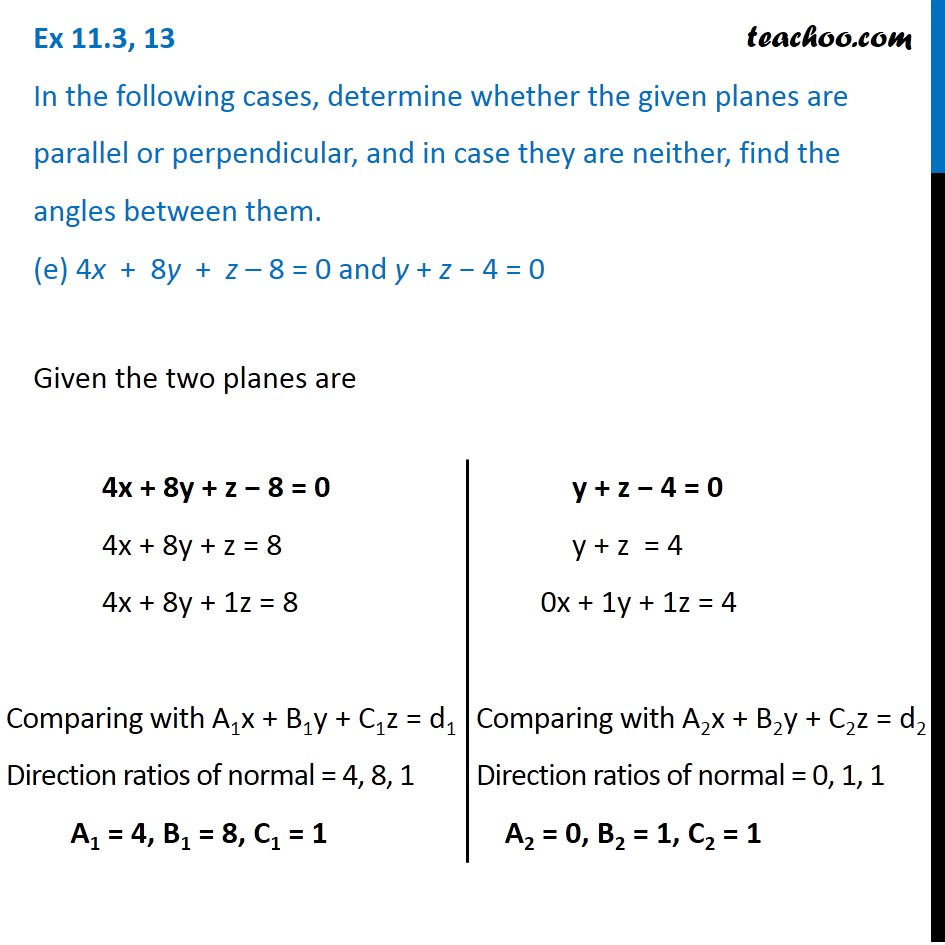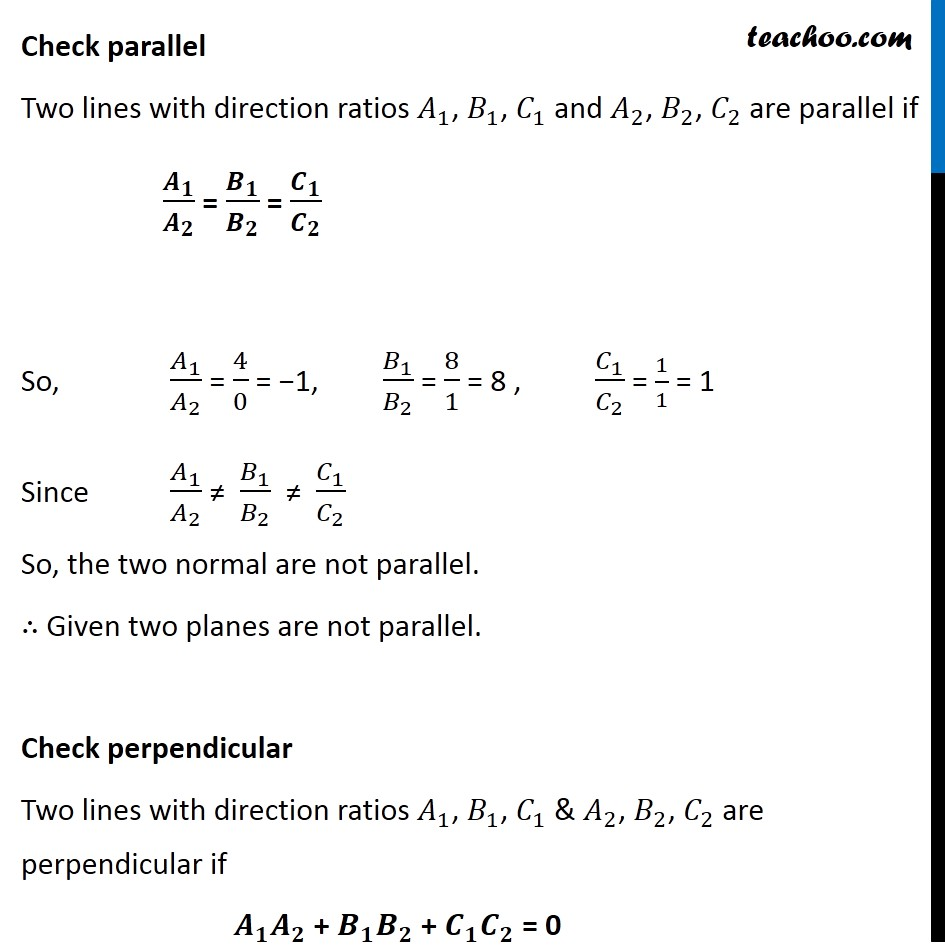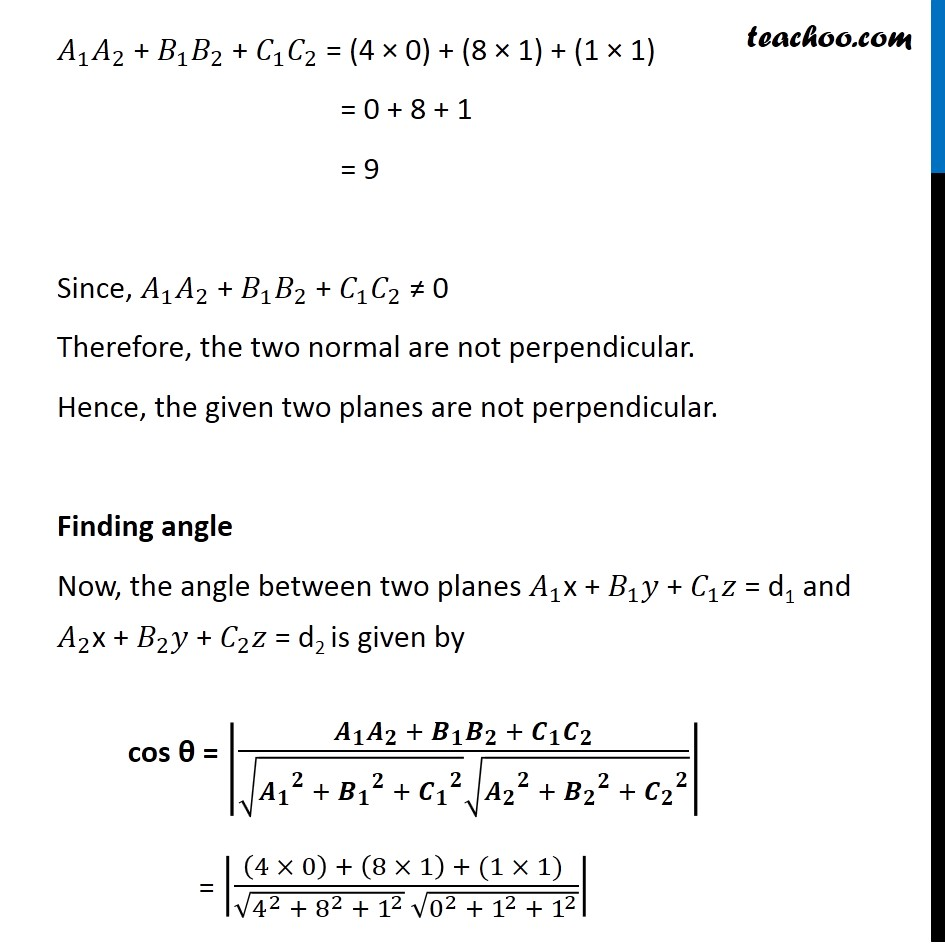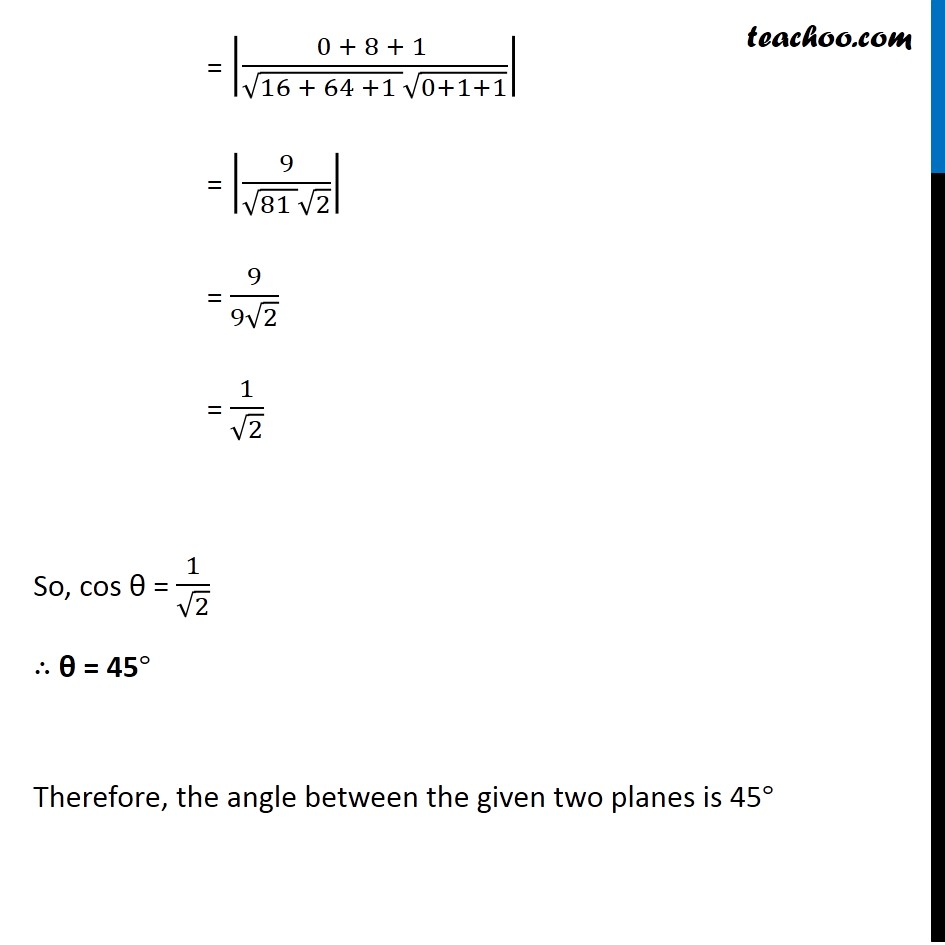Plane

Chapter 11 Class 12 Three Dimensional Geometry
Serial order wiseLearn in your speed, with individual attention - Teachoo Maths 1-on-1 Class

### Transcript

Question 13 In the following cases, determine whether the given planes are parallel or perpendicular, and in case they are neither, find the angles between them. (e) 4x + 8y + z – 8 = 0 and y + z − 4 = 0 4x + 8y + z − 8 = 0 4x + 8y + z = 8 4x + 8y + 1z = 8 Comparing with A1x + B1y + C1z = d1 Direction ratios of normal = 4, 8, 1 A1 = 4, B1 = 8, C1 = 1 y + z − 4 = 0 y + z = 4 0x + 1y + 1z = 4 Comparing with A2x + B2y + C2z = d2 Direction ratios of normal = 0, 1, 1 A2 = 0, B2 = 1, C2 = 1 Check parallel Two lines with direction ratios 𝐴_1, 𝐵_1, 𝐶_1 and 𝐴_2, 𝐵_2, 𝐶_2 are parallel if 𝑨_𝟏/𝑨_𝟐 = 𝑩_𝟏/𝑩_𝟐 = 𝑪_𝟏/𝑪_𝟐 So, 𝐴_1/𝐴_2 = 4/0 = −1, 𝐵_1/𝐵_2 = 8/1 = 8 , 𝐶_1/𝐶_2 = 1/1 = 1 Since 𝐴_1/𝐴_2 ≠ 𝐵_1/𝐵_2 ≠ 𝐶_1/𝐶_2 So, the two normal are not parallel. ∴ Given two planes are not parallel. Check perpendicular Two lines with direction ratios 𝐴_1, 𝐵_1, 𝐶_1 & 𝐴_2, 𝐵_2, 𝐶_2 are perpendicular if 𝑨_𝟏 𝑨_𝟐 + 𝑩_𝟏 𝑩_𝟐 + 𝑪_𝟏 𝑪_𝟐 = 0 𝐴_1 𝐴_2 + 𝐵_1 𝐵_2 + 𝐶_1 𝐶_2 = (4 × 0) + (8 × 1) + (1 × 1) = 0 + 8 + 1 = 9 Since, 𝐴_1 𝐴_2 + 𝐵_1 𝐵_2 + 𝐶_1 𝐶_2 ≠ 0 Therefore, the two normal are not perpendicular. Hence, the given two planes are not perpendicular. Finding angle Now, the angle between two planes 𝐴_1x + 𝐵_1 𝑦 + 𝐶_1 𝑧 = d1 and 𝐴_2x + 𝐵_2 𝑦 + 𝐶_2 𝑧 = d2 is given by cos θ = |(𝑨_𝟏 𝑨_𝟐 + 𝑩_𝟏 𝑩_𝟐 + 𝑪_𝟏 𝑪_𝟐)/(√(〖𝑨_𝟏〗^𝟐 + 〖𝑩_𝟏〗^𝟐 + 〖𝑪_𝟏〗^𝟐 ) √(〖𝑨_𝟐〗^𝟐 + 〖𝑩_𝟐〗^𝟐 + 〖𝑪_𝟐〗^𝟐 ))| = |((4 × 0) + (8 × 1) + (1 × 1) )/(√(4^2 + 8^2 + 1^2 ) √(0^2 + 1^2 + 1^2 ))| = |(0 + 8 + 1 )/(√(16 + 64 +1 ) √(0+1+1))| = |9/(√(81 ) √2)| = 9/(9√2) = 1/√2 So, cos θ = 1/√2 ∴ θ = 45° Therefore, the angle between the given two planes is 45°